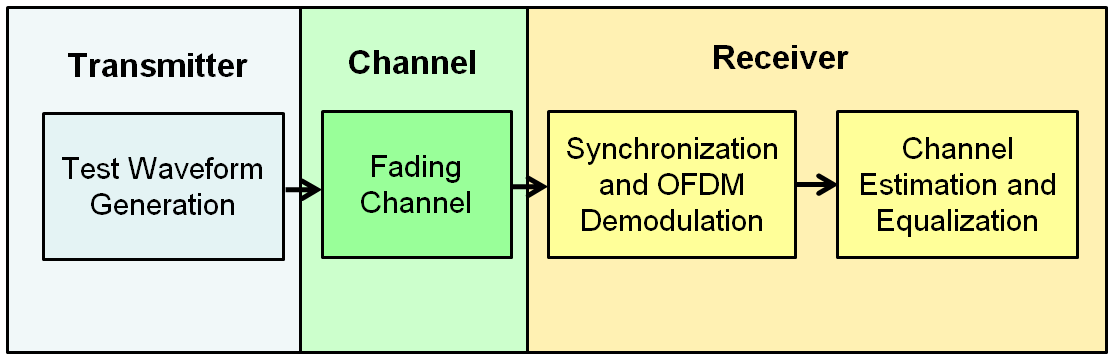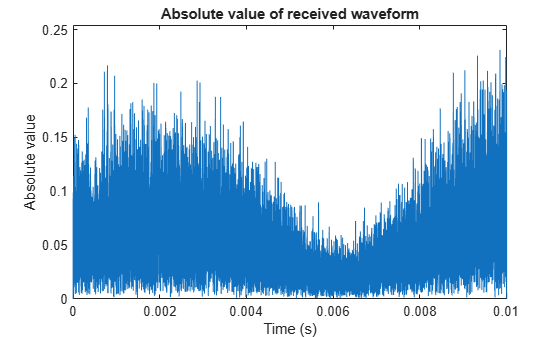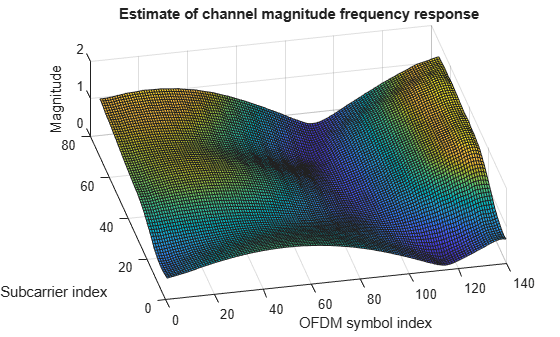This example shows how to implement an LTE transmit and receive chain, as shown in this figure.Generate an E-UTRA test model (E-TM) configuration. Use this configuration to generate the waveform and populate the resource grid.

enb = lteTestModel('1.1','1.4MHz');
[txwave,txgrid,info] = lteTestModelTool(enb);

Plot a graphical representation of the transmit resource grid.

figure('Color','w');
helperPlotTransmitResourceGrid(enb,txgrid);The figure shows a resource grid populated with E-TM 1.1 resource elements.

Simulate transmission through a fading channel propagation model.

channel.ModelType = 'GMEDS';
channel.DelayProfile = 'EVA';
channel.DopplerFreq = 70;
channel.MIMOCorrelation = 'Medium';
channel.NRxAnts = 1;
channel.InitTime = 0;
channel.InitPhase = 'Random';
channel.Seed = 17;
channel.NormalizePathGains = 'On';
channel.NormalizeTxAnts = 'On';
channel.SamplingRate = info.SamplingRate;
channel.NTerms = 16;

Plot the time-varying power of the received waveform.

figure('Color','w');This plot shows the waveform power variation over time.

Perform frame synchronization.

offset = lteDLFrameOffset(enb,rxwave);
rxwave = rxwave(offset:end,:);

Perform OFDM demodulation.

rxgrid = lteOFDMDemodulate(enb,rxwave);

Create a surface plot showing the power of the received grid for each subcarrier and OFDM symbol.

figure('Color','w');This plot shows the received grid power.

Estimate the channel and noise.

cec.PilotAverage = 'UserDefined';
cec.FreqWindow = 9;
cec.TimeWindow = 9;
cec.InterpType = 'Cubic';
cec.InterpWindow = 'Centered';
cec.InterpWinSize = 3;
[hest,nest] = lteDLChannelEstimate(enb,cec,rxgrid);

Create a surface plot showing the magnitude of the channel estimate for each OFDM symbol across the subcarriers.

figure('Color','w');
helperPlotChannelEstimate(hest);This figure shows an estimate of channel magnitude frequency response.

Finally, perform minimum mean-square error (MMSE) equalization on the received grid.

eqgrid = lteEqualizeMMSE(rxgrid,hest,nest);

Create a surface plot of the power of the equalized resource grid, in dB.

figure('Color','w');
helperPlotEqualizedResourceGrid(enb,eqgrid);As can be seen the equalization flattened the power response across the resource grid.